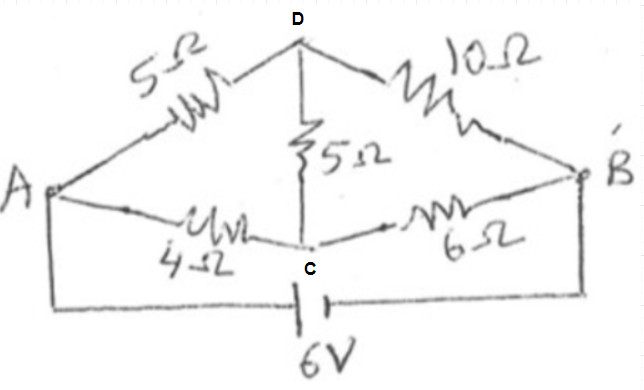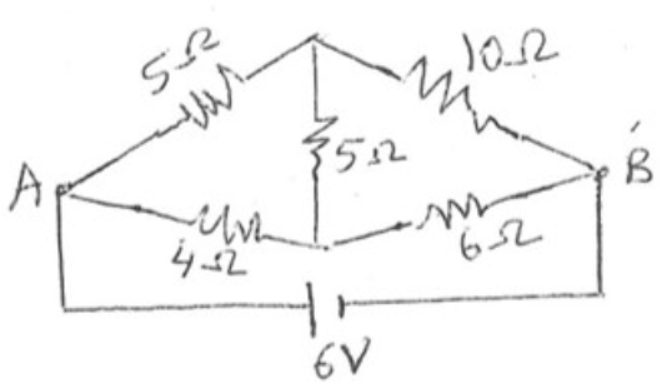# Problem: (a) What is the current through the battery in the circuit shown below? (b) What is the equivalent resistance between A and B for the network of resistors?

###### FREE Expert Solution

Consider relabeling:Current:

$\overline{){\mathbf{I}}{\mathbf{=}}\frac{\mathbf{V}}{\mathbf{R}}}$

79% (451 ratings)###### Problem Details

(a) What is the current through the battery in the circuit shown below?

(b) What is the equivalent resistance between A and B for the network of resistors?